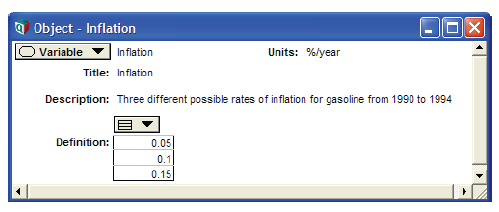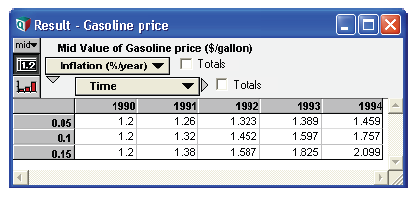# Dynamic using arrays

The initial value of a dynamic variable — that is, the first parameter to the Dynamic function — can be a number, variable identifier, or other expression that evaluates to a single number, list, or array. Analytica evaluates a dynamic variable starting from each initial value, in each time period, so the result is a correctly dimensioned array.

Example: Expanding the example (see Dynamic function), suppose the inflation rate of gasoline is uncertain. Instead of providing a single numerical value, you could define the inflation rate as a list.Using the new Inflation variable in the definition for Gasprice, the results show three different rates of increases in gasoline prices from 1990 to 1994:

Variable Gasprice :=
Dynamic(1.2, Gasprice[Time - 1]*(1 + Inflation)) →